﻿ 基于激光测距仪的旋翼飞行器位置估计算法 Position Estimation Algorithm for Rotorcraft Based on Laser Range Finder

Dynamical Systems and Control
Vol.06 No.04(2017), Article ID:22551,8 pages
10.12677/DSC.2017.64024

Position Estimation Algorithm for Rotorcraft Based on Laser Range Finder

*通讯作者。

Guifang Tan, Chenxiao Cai*

School of Automation, Nanjing University of Science and Technology, Nanjing Jiangsu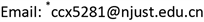Received: Oct. 2nd, 2017; accepted: Oct. 23rd, 2017; published: Oct. 31st, 2017ABSTRACT

In this paper, the environment information is captured and the position of rotorcraft is calculated by using laser scanner and using iterative closest point (ICP) algorithm. It could estimate the position more rapid and accurate by improving the extended Kalman filter (EKF) algorithm which reduces the amount of computation. The trajectory model of rotorcraft will be constructed by combining and fusing the ICP and improved EKF algorithms to update the prediction. In the process of updating, the observed environment model will be sketched through extracting line features for surrounding of the rotorcraft. Then the model expression is simplified according to the accurate attitude angles of rotorcraft which come from Inertial Measurement Unit (IMU) sensor. Finally, location information is estimated accurately for the rotorcraft via simulation and experiment.

Keywords:Rotorcraft, Laser Scanner, ICP Algorithm, EKF Algorithm, Position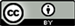1. 引言

2. 坐标系的建立

2.1. 机体坐标系

$\left\{\begin{array}{c}{x}_{i}^{b}={d}_{i}\mathrm{cos}\left({\phi }_{i}-\text{π}/4\right)\\ {y}_{i}^{b}={d}_{i}\mathrm{sin}\left({\phi }_{i}-\text{π}/4\right)\end{array}$ (1)

${x}_{b}$ , ${y}_{b}$ 是机体坐标系下x, y的坐标值。

2.2. 大地坐标系

$\left\{\begin{array}{c}{x}_{i}^{w}={x}_{i}^{b}\mathrm{cos}\theta -{y}_{i}^{b}\mathrm{sin}\theta \\ {y}_{i}^{w}={x}_{i}^{b}\mathrm{sin}\theta +{y}_{i}^{b}\mathrm{cos}\theta \end{array}$ (2)

${x}_{w}$ , ${y}_{w}$ 表示大地坐标系下的x, y的坐标值(图2)。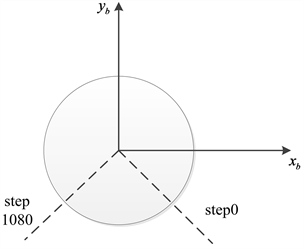Figure 1. Curve: sketch map of body coordinate axis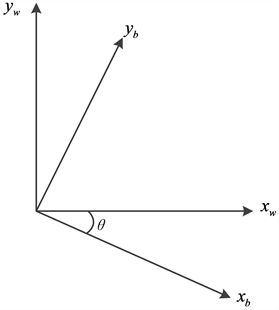Figure 2. Curve: The relation between body coordinate and ground coordinate

3. 环境中特征线段的提取

$P=\left\{{S}_{1},{S}_{2},{S}_{3},\cdots ,{S}_{M}\right\}$

(1) 根据实际需要，确定一个点和线段关系的阈值。

(2) 点集 ${S}_{i}$ 作为待处理数据点，连接待处理数据点的起始点和终止点，建立一个线段的模型。

(3) 找到待处理数据点中其它数据点距离线段的距离，记录其最大值，判断这个最大值与阈值的关系，如果最大值小于阈值，则不进行分割，否则，把线段分为两部分。

(4) 判断点集 ${S}_{i}$ 中的线段是否处理完，如果没有，跳到第(2)步。

4. 改进的EKF算法

4.1. 特征表示与选择

4.2. 模型的建立

4.2.1. 观测模型的建立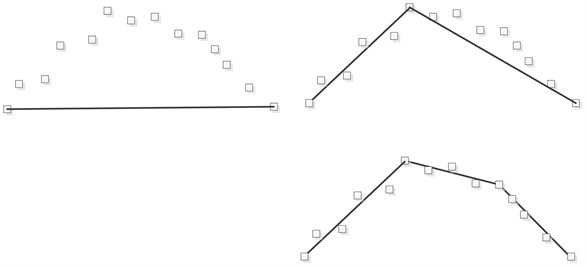Figure 3. Curve: Sketch map of line segment extraction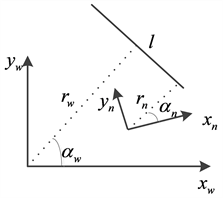Figure 4. Curve: the definition of line in body and ground coordinates, respectively

${h}_{i}\left(X,M\right)=\left(\begin{array}{c}{r}_{i}-\sqrt{{x}^{2}+{y}^{2}}\mathrm{cos}\left(\beta -{\alpha }_{i}\right)\\ {\alpha }_{i}-\theta \end{array}\right)$ (3)

${h}_{i}\left(X,M\right)=\left({r}_{i}-\sqrt{{x}^{2}+{y}^{2}}\mathrm{cos}\left(\beta -{\alpha }_{i}\right)\right)$ (4)

${H}_{i}=\left[\begin{array}{cc}{H}_{11}& {H}_{12}\end{array}\right]$ (5)

${H}_{11}=-\frac{x}{\rho }\mathrm{cos}\left(\beta -{\alpha }_{i}\right)-\frac{y}{\rho }\mathrm{sin}\left(\beta -{\alpha }_{i}\right)$

${H}_{12}=-\frac{y}{\rho }\mathrm{cos}\left(\beta -{\alpha }_{i}\right)+\frac{x}{\rho }\mathrm{sin}\left(\beta -{\alpha }_{i}\right)$

$\rho =\sqrt{{x}^{2}+{y}^{2}}$

$\left\{\begin{array}{l}{K}_{k,i}={P}_{k|k,i-1}{H}_{i}^{T}\left({H}_{i}{P}_{k|k,i-1}{H}_{i}^{\text{T}}+{R}_{i}\right)\hfill \\ {x}_{k|k-1,i}={x}_{k|k-1,i}+{K}_{k,i}\left({Y}_{i}-{h}_{i}\left(X,M\right)\right)\hfill \\ {P}_{k|k,i}=\left(I-{K}_{k,i}{H}_{i}\right){P}_{k|k,i-1}\hfill \end{array}$ (6)

4.2.2. 过程模型的建立

$\left\{\begin{array}{c}{x}_{k|k-1}={x}_{k-1|k-1}+\mathrm{cos}{\theta }_{k}\Delta x-\mathrm{sin}{\theta }_{k}\Delta y\\ {y}_{k|k-1}={y}_{k-1|k-1}+\mathrm{sin}{\theta }_{k}\Delta x+\mathrm{cos}{\theta }_{k}\Delta y\end{array}$ (7)

5. 实验结果分析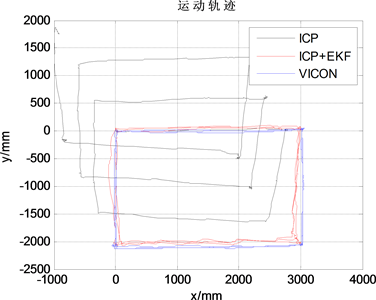Figure 5. Curve: Trajectory map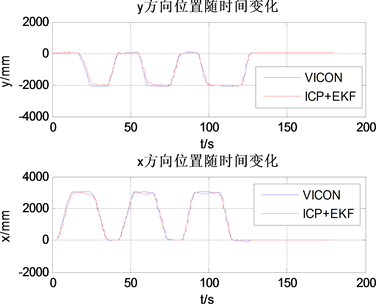Figure 6. Curve: The position of the x direction and the position of the y direction versus time

6. 结论

Position Estimation Algorithm for Rotorcraft Based on Laser Range Finder[J]. 动力系统与控制, 2017, 06(04): 187-194. http://dx.doi.org/10.12677/DSC.2017.64024

1. 1. 段世华. 四旋翼飞行器控制系统的设计和实现[D]: [硕士学位论文]. 北京: 电子科技大学, 2012.

2. 2. 吴中杰. 四旋翼飞行器设计及其姿态控制[D]: [硕士学位论文]. 南京:东南大学, 2012.

3. 3. Mao, G., Drake, S. and Anderson, B.D.O. (2007) Design of an Extended Kalman Filter for UAV Localization. Information, Decision and Control, 224-229. https://doi.org/10.1109/IDC.2007.374554

4. 4. Lange, S., Sunderhauf, N. and Protzel, P. (2009) A Vision Based Onboard Approach for Landing and Position Control of an Autonomous Multirotor UAV in GPS-Denied Environments. International Conference on Advanced Robotics, 1-6.

5. 5. 隋婧, 金伟其. 双目立体视觉技术的实现及其进展[J]. 电子技术应用, 2004, 30(10): 4-6.

6. 6. Borenstein, J. and Koren, Y. (2002) The Vector Field Histogram-Fast Obstacle Avoidance for Mobile Robots. IEEE Transactions on Robotics & Automation, 7, 278-288. https://doi.org/10.1109/70.88137

7. 7. 任立敏. 基于激光测距仪的移动机器人障碍感知与避障策略研究[D]: [硕士学位论文]. 哈尔滨: 哈尔滨工业大学, 2007.

8. 8. 彭晟远. 基于激光测距仪的室内机器人SLAM研究[D]: [硕士学位论文]. 武汉: 武汉科技大学, 2012.

9. 9. 徐晓东. 移动机器人几何–拓扑混合地图构建及定位研究[D]: [硕士学位论文]. 大连: 大连理工大学, 2005.

10. 10. Luo, C., Mcclean, S.I., Parr, G., et al. (2013) UAV Position Es-timation and Collision Avoidance Using the Extended Kalman Filter. IEEE Transactions on Vehicular Technology, 62, 2749-2762. https://doi.org/10.1109/TVT.2013.2243480

11. 11. Li, R., Liu, J. and Zhang, L. (2014) LIDAR/MEMS IMU Inte-grated Navigation (SLAM) Method for a Small UAV in Indoor Environments. Inertial Sensors and Systems Symposium, Karlsruhe, 16-17 September 2014, 1-15. https://doi.org/10.1109/InertialSensors.2014.7049479

12. 12. 祝继华, 郑南宁, 袁泽剑, 等. 基于ICP算法和粒子滤波的未知环境地图创建[J]. 自动化学报, 2009, 35(8): 1107-1113.

13. 13. Hiremath, S.A., van der Heijden, G.W.A.M., Evert, F.K.V., et al. (2014) Laser Range Finder Model for Autonomous Navigation of a Robot in a Maize Field Using a Particle Filter. Computers & Electronics in Agriculture, 100, 41-50. https://doi.org/10.1016/j.compag.2013.10.005

14. 14. Grisetti, G., Kummerle, R., Stachniss, C., et al. (2011) A Tu-torial on Graph-Based SLAM. IEEE Intelligent Transportation Systems Magazine, 2, 31-43. https://doi.org/10.1109/MITS.2010.939925

15. 15. Daum, F. (2005) Nonlinear Filters: Beyond the Kalman Filter. IEEE Aerospace & Electronic Systems Magazine, 20, 57-69. https://doi.org/10.1109/MAES.2005.1499276

16. 16. 曲仕茹, 马志强. 改进的粒子滤波在四旋翼姿态估计中的应用[J]. 飞行力学, 2013, 31(5): 458-461.

17. 17. Cui, J. (2014) Navigation of Unmanned Aerial Vehicles in GPS-Denied Environments. Ph.D. Thesis, National University of Singapore, Singapore, 92-104.

18. 18. 王龙, 章政, 王立. 改进扩展卡尔曼滤波的四旋翼姿态估计算法[J]. 计算机应用, 2017, 37(4): 1122-1128.

19. 19. Perera, A.G.A., Srinivas, C., Hoogs, A., et al. (2006) Multi-Object Tracking through Simultaneous Long Occlusions and Split-Merge Conditions. 2006 IEEE Computer Society Conference, New York, 17-22 June 2006, 666-673. https://doi.org/10.1109/CVPR.2006.195

20. 20. Jensfelt, P. and Christensen, H.I. (2001) Pose Tracking Using Laser Scanning and Minimalistic Environmental Models. IEEE Transactions on Robotics & Automation, 17, 138-147. https://doi.org/10.1109/70.928559